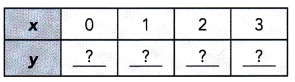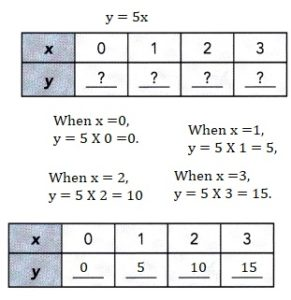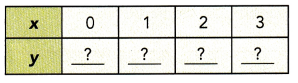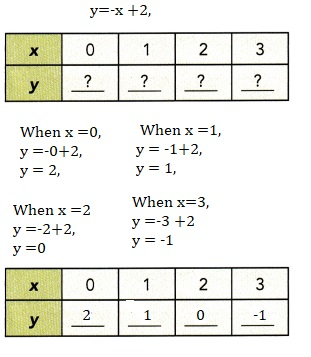# Math in Focus Grade 8 Chapter 5 Answer Key Systems of Linear Equations

Practice the problems of Math in Focus Grade 8 Workbook Answer Key Chapter 5 Systems of Linear Equations to score better marks in the exam.

## Math in Focus Grade 8 Course 3 A Chapter 5 Answer Key Systems of Linear Equations

### Math in Focus Grade 8 Chapter 5 Quick Check Answer Key

Complete the table of values and graph each linear equation.

Question 1.
y = 5xWhen x = 0, y=0,
x =1 , y = 5, x = 2, y = 10, when x = 3 y =15,

Explanation:Question 2.
y = -x + 2x =0, y = 2, x= 1, y =1,
x= 2, y = 0, x =3,y =-1,

Explanation:Question 3.
Samuel bought 30 books. The hardcover books cost $20 each while the rest, which are paperbacks, cost$8 each. If he spent a total of \$480, how many paperbacks did he buy?